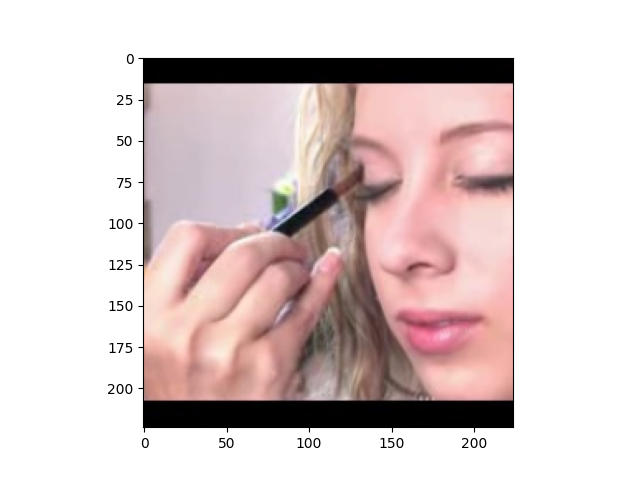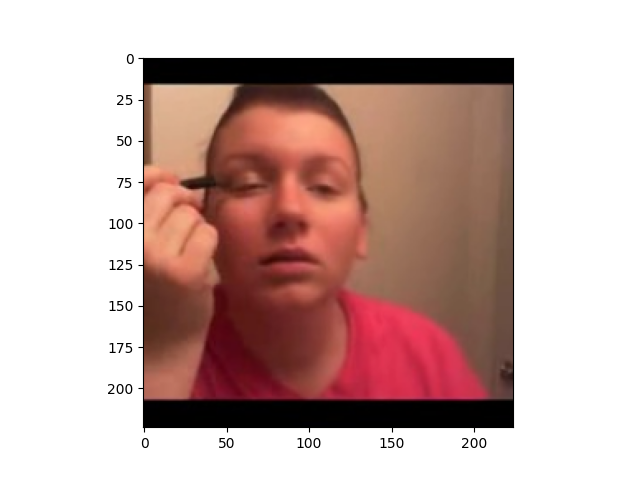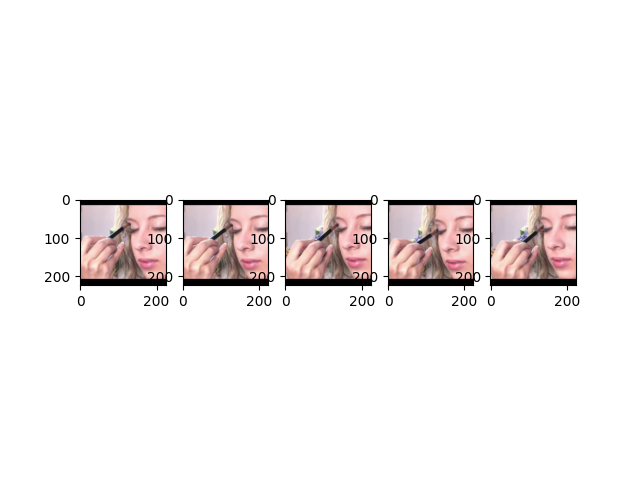# Prepare the UCF101 dataset¶

UCF101 is an action recognition dataset of realistic action videos, collected from YouTube. With 13,320 short trimmed videos from 101 action categories, it is one of the most widely used dataset in the research community for benchmarking state-of-the-art video action recognition models. This tutorial will go through the steps of preparing this dataset for GluonCV.## Setup¶

We need the following two files from UCF101: the dataset and the official train/test split.

Filename

Size

UCF101.rar

6.5 GB

114 KB

The easiest way to download and unpack these files is to download helper script `ucf101.py` and run the following command:

```python ucf101.py
```

This script will help you download the dataset, unpack the data from compressed files, decode the videos to frames, and generate the training files for you. All the files will be stored at `~/.mxnet/datasets/ucf101` by default.

Note

You need at least 60 GB disk space to download and extract the dataset. SSD (Solid-state disks) is preferred over HDD because of faster speed.

You may need to install `unrar` by `sudo apt install unrar`.

You may need to install `rarfile`, `Cython`, `mmcv` by `pip install rarfile Cython mmcv`.

The data preparation process may take a while. The total time to prepare the dataset depends on your Internet speed and disk performance. For example, it takes about 30min on an AWS EC2 instance with EBS.

The prepared dataset can be loaded with utility class `gluoncv.data.UCF101` directly. In this tutorial, we provide three examples to read data from the dataset, (1) load one frame per video; (2) load one clip per video, the clip contains five consecutive frames; (3) load three clips evenly per video, each clip contains 12 frames.

We first show an example that randomly reads 25 videos each time, randomly selects one frame per video and performs center cropping.

```from gluoncv.data import UCF101
from mxnet.gluon.data.vision import transforms
from gluoncv.data.transforms import video

transform_train = transforms.Compose([
video.VideoCenterCrop(size=224),
video.VideoToTensor()
])

# Default location of the data is stored on ~/.mxnet/datasets/ucf101.
# You need to specify ``setting`` and ``root`` for UCF101 if you decoded the video frames into a different folder.
train_dataset = UCF101(train=True, transform=transform_train)
```

We can see the shape of our loaded data as below. `extra` indicates if we select multiple crops or multiple segments from a video. Here, we only pick one frame per video, so the `extra` dimension is 1.

```for x, y in train_data:
print('Video frame size (batch, extra, channel, height, width):', x.shape)
print('Video label:', y.shape)
break
```

Out:

```Video frame size (batch, extra, channel, height, width): (25, 1, 3, 224, 224)
Video label: (25,)
```

Let’s plot several training samples. index 0 is image, 1 is label

```from gluoncv.utils import viz
viz.plot_image(train_dataset.squeeze().transpose((1,2,0))*255.0)  # CricketBowling
```
••Here is the second example that randomly reads 25 videos each time, randomly selects one clip per video and performs center cropping. A clip can contain N consecutive frames, e.g., N=5.

```train_dataset = UCF101(train=True, new_length=5, transform=transform_train)
```

We can see the shape of our loaded data as below. Now we have another `depth` dimension which indicates how many frames in each clip (a.k.a, the temporal dimension).

```for x, y in train_data:
print('Video frame size (batch, extra, channel, depth, height, width):', x.shape)
print('Video label:', y.shape)
break
```

Out:

```Video frame size (batch, extra, channel, depth, height, width): (25, 1, 3, 5, 224, 224)
Video label: (25,)
```

Let’s plot one training sample with 5 consecutive video frames. index 0 is image, 1 is label

```from matplotlib import pyplot as plt
# subplot 1 for video frame 1
fig = plt.figure()
frame1 = train_dataset[0,:,0,:,:].transpose((1,2,0)).asnumpy()*255.0
plt.imshow(frame1.astype('uint8'))
# subplot 2 for video frame 2
frame2 = train_dataset[0,:,1,:,:].transpose((1,2,0)).asnumpy()*255.0
plt.imshow(frame2.astype('uint8'))
# subplot 3 for video frame 3
frame3 = train_dataset[0,:,2,:,:].transpose((1,2,0)).asnumpy()*255.0
plt.imshow(frame3.astype('uint8'))
# subplot 4 for video frame 4
frame4 = train_dataset[0,:,3,:,:].transpose((1,2,0)).asnumpy()*255.0
plt.imshow(frame4.astype('uint8'))
# subplot 5 for video frame 5
frame5 = train_dataset[0,:,4,:,:].transpose((1,2,0)).asnumpy()*255.0
plt.imshow(frame5.astype('uint8'))
# display
plt.show()
```The last example is that we randomly read 25 videos each time, select three clips evenly per video and performs center cropping. A clip contains 12 consecutive frames.

```train_dataset = UCF101(train=True, new_length=12, num_segments=3, transform=transform_train)
```

We can see the shape of our loaded data as below. Now the `extra` dimension is 3, which indicates we have three segments for each video.

```for x, y in train_data:
print('Video frame size (batch, extra, channel, depth, height, width):', x.shape)
print('Video label:', y.shape)
break
```

Out:

```Video frame size (batch, extra, channel, depth, height, width): (25, 3, 3, 12, 224, 224)
Video label: (25,)
```